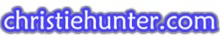Home About Me disabled former page Arthur Articles

# Statistics

The following represents Arthur's opinions only and not necessarily those of Christie.

 Computer Help Personal Music Statistics Pain Pathophysiology Theory of Personality

SAT SUMX = 5846, SUMX^2 = 3428148

A) Mean = 5846 / 10 = 584.6

B) S = ((SUMX^2-(((SUMX)^2)/N))/(N-1))^.5

S = ((3428148-(((5846)^2)/10))/(10-1))^.5

S = ((3428148-3417571.6)/9)^.5

S = 1175.12^.5

S = 34.28

C) 95%CI = +- t(.05)SsubXBAR + XBAR

SSubXBAR = (s)/(n^.5) = 34.28/3.16 = 10.85

95%CI = +- t(.05)10.85 + 584.6 = +-(2.2622*10.85)+ 584.6

95%CI = 560.07 - 609.13 or the whole scores of 560-609.  542 is not in the expected range of the population mean, thus the course has a statistical significance of improving the scores.

By using a confidence interval of 95%, we can be 95% sure that the population mean is contained within 560.06 - 609.14.   Since a mean of 542 is not within this range, we have can conclude that the new course did indeed improve the SAT verbal scores.

By performing a one-tailed t test we can analyze this:

Set H0: u0<=u

Set H1: u>u0

in plain language:  our null hypothesis is that the sample mean is equal to or less than the population mean.  Our alternative hypothesis is that the sample mean is greater than the population mean.t = (584.6-542)/(10.841) = 3.93

t(.01),df=9 = 2.82, p<.01

Rejection Rule:   For H1:m > m0, reject H0 if tcomp > tcrit

We reject H0.

The new course did improved the SAT verbal scores

Question #2

Descriptive Statistics

 N Mean Std. Deviation Variance Yellow 5 20.6000 4.39318 19.300 Green 4 29.2500 12.09339 146.250 Valid N (listwise) 4

Our Null hypothesis:  H0 = u1 - u2 = 0 (there is no difference in the scores of the yellow group and green group)

Our alpha level is .05, we are looking for a 95% CI

Since N is different for each group, we use the formula:

s sub Xbar sub 1 minus X bar sub 2 = ((((N1-1)(s2sub1) + (N2-1)(S2sub2))/(N1+N2-2))((1/N1)+(1/N2)))^.5

OR: (s2pooled(1/N1 + 1/N2))^.5

S2sub1 = 19.3

S2sub2 = 146.25

N1=5

N2=4

So we get: s2pooled = ((4*19.3)+(3*146.25))/7

s2pooled = 515.95/7 = 73.71

s sub Xbar sub 1 minus X bar sub 2 = (73.71(1/5 + 1/4))^.5 = 5.76, or the standard error difference

Now find t sub xbar sub 1 minus xbar sub 2 = (Xbar1 - Xbar2)/s sub Xbar sub 1 minus X bar sub 2

=20.60-29.25/5.76 = -1.50

Using table B, we find a critical value for T where df = N1+N2-2, or 7

Using the 5% level for t(.05) we get 2.36

Rejection Rule:  Reject H0 if |tcomp| >= tcrit

|tcomp| = 1.5 which is less than 2.36, or tcrit, therefore we do not reject H0, meaning that we cannot conclude the yellow paper caused subjects to score lower.  The answer is no

T-Test, Question 2

Group Statistics

 Color N Mean Std. Deviation Std. Error Mean Value Yellow 5 20.6000 4.39318 1.96469 Green 4 29.2500 12.09339 6.04669

Independent Samples Test

 Levene's Test for Equality of Variances t-test for Equality of Means F Sig. t df Sig. (2-tailed) Mean Difference Std. Error Difference 95% Confidence Interval of the Difference Lower Upper Value 3.385 .108 -1.502 7 .177 -8.65000 5.75919 -22.26831 4.96831

Equal variances assumed

Question #3                         Statistics

 DietA DietB DietC N 8 10 10 Mean 8.1250 9.0000 10.7000 Median 8.0000 9.0000 11.0000 Mode 7.00(a) 9.00 7.00(a) Std. Deviation 2.35660 2.30940 2.75076 Variance 5.554 5.333 7.567

a  Multiple modes exist. The smallest value is shown

For Diet A, the most appropriate descriptive statistic is the mean.  Since multiple modes exist, the mode is not very useful.  The median is slightly lower than the mean, giving it a slight positive skew, hence the mean is the most useful.

For Diet B, the median, mean and mode are all equal, hence all useful.

For Diet C, there is a slight negative skew.  Multiple modes exist, so the mode is not useful.  Because the skew is only slight, the mean is the most useful.

Histograms are the best graphs for these diets.

Graph of Diet A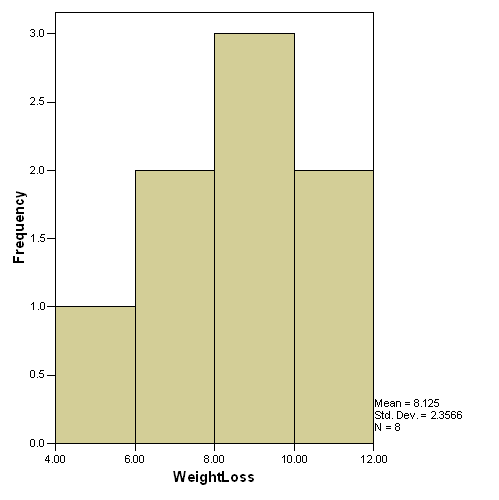Diet B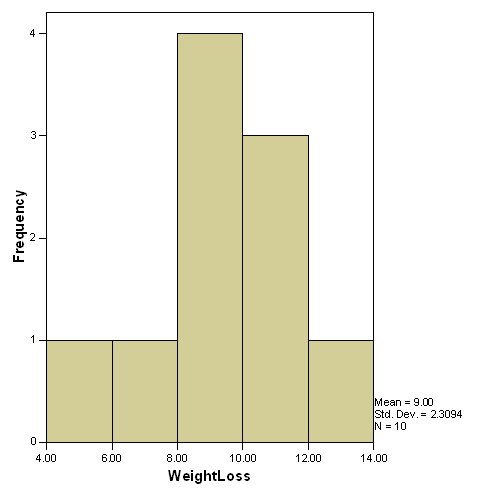Diet C#3, Oneway ANOVA

Build a matrix of values based on the data for Diet A, Diet B, Diet C

Ho: m1 = m2 = m3

Ha: At least one mean differs from the others.

Rejection Rule:  Reject Ho if p-value < .05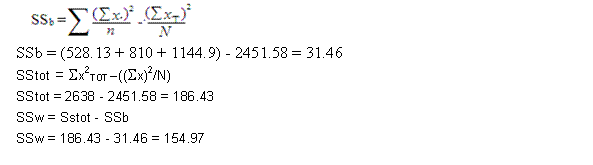SPSS ANOVA

Weight_Loss

 Sum of Squares df Mean Square F Sig. Between Groups 31.454 2 15.727 2.537 .099 Within Groups 154.975 25 6.199 Total 186.429 27

P value = .099 > .05 --> Accept H0.

F(2,25) = 3.39 , p = .099

There is no significant difference in mean wieght loss among the different diets

#3 b) Post Hoc Tests

Multiple Comparisons

Dependent Variable: Weight_Loss

LSD

 (I) Diet_Type (J) Diet_Type Mean Difference (I-J) Std. Error Sig. 95% Confidence Interval Lower Bound Upper Bound Diet A Diet B -.87500 1.18101 .466 -3.3073 1.5573 Diet C -2.57500(*) 1.18101 .039 -5.0073 -.1427 Diet B Diet A .87500 1.18101 .466 -1.5573 3.3073 Diet C -1.70000 1.11346 .139 -3.9932 .5932 Diet C Diet A 2.57500(*) 1.18101 .039 .1427 5.0073 Diet B 1.70000 1.11346 .139 -.5932 3.9932

*  The mean difference is significant at the .05 level.

There was a significant difference between Diet A and Diet C

#3 b) A graph of the means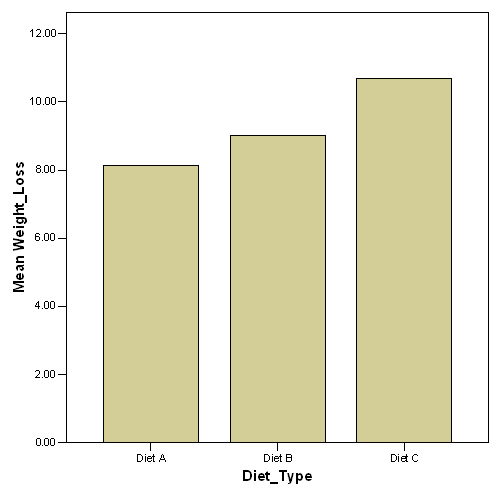#4, Two Way ANOVA, Type I Sum of Squares

A

B

C

M

Sx11

28

Sx12

57

Sx13

38

SA1

123

Sx211

210

Sx212

547

Sx213

490

SA21

124779.5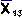12.667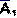9.462

n11

4

n12

6

n13

3

nA1

13

F

Sx21

37

Sx22

28

Sx23

76

SA2

141

Sx221

357

Sx222

206

Sx223

870

SA22

1433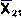9.25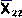710.857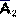9.4

n21

4

n22

4

n23

7

nA2

15

SB1

65

SB2

85

SB3

114

SXT

264

SB21

567

SB22

753

SB23

1360

SX2T

26808.1258.5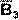11.4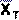9.429

nB1

8

nB2

10

nB3

10

N

28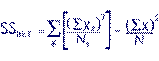SSbet = (28^2/4)+(57^2/6)+(38^2/3)+(37^2/4)+(28^2/4)+(76^2/7)-(264^2/28)

= 196+541.5+481.333+342.25+196+825.143-2489.143 = 93.083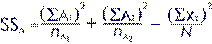SSa = (123^2/13)+(141^2/15)-(264^2/28)

= 1163.769+1325.4 - 2489.143 = 0.026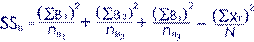= (65^2/8)+(85^2/10)+(114^2/10)-(264^2/28)

= 528.125+722.5+1299.6-2489.143 = 61.082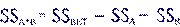SSa*b = 93.083-0.026-61.082 = 31.975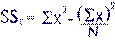SSt = 2680 - (264^2)/28 = 2680 - 2489.14285714286 = 190.857

SSw = SSt - SSbet

= 190.857 - 93.083 = 97.774

dfbet = K - 1 = 5

K = total number of groups

dfa = A - 1 = 1

A = #of cells in A

dfb = B - 1 = 2

B = #of cells in B

dft = N - 1 = 28-1 = 27

dfa*b = 1*2 = 2

MSbet = SSbet/dfbet = 93.083/5 = 18.617

MSa = SSa/dfa = 0.026/1 = 0.026

MSb = SSb/dfb = 61.082/2 = 30.541

MSa*b = Ssa*b/dfa*b = 31.975/2 = 15.988

MSw = SSw/dfw = 97.774/22 = 4.444

Fa = MSa/MSw = 0.026/4.444 = 0.006 (df=1,22)

Fb = MSb/MSw = 30.541/4.444 = 6.872 (df=2,22)

Fa*b = Msa*b/MSw = 15.988/ = 3.598 (df=2,22)

 Two-Way ANOVA Summary Table Source SS df MS F p Between Groups (Cells) 93.08 5 18.62 A 0.026 1 0.026 0.006 (df=1,22) F(1,22)=0.006, p>.05 B 61.08 2 30.54 6.872 (df=2,22) F(2,22)=6.872, p<.01 A x B 31.98 2 15.99 3.598 (df=2,22) F(2,22)=3.598, p<.05 Within Groups 97.77 22 4.444 Total 190.9 27

F(1,22)=0.006, p>.05

There is no significant population mean difference among Gender

F(2,22)=6.872, p<.01

There is a significant population mean difference in the diets

F(2,22)=3.598, p<.05

There is a significant interaction between diets and gender

Tests of Between-Subjects Effects

Dependent Variable: Weight_Loss

 Source Type I Sum of Squares df Mean Square F Sig. Corrected Model 93.083(a) 5 18.617 4.189 .008 Intercept 2489.143 1 2489.143 560.080 .000 Gender .026 1 .026 .006 .939 Diet 65.377 2 32.689 7.355 .004 Gender * Diet 27.680 2 13.840 3.114 .064 Error 97.774 22 4.444 Total 2680.000 28 Corrected Total 190.857 27

a  R Squared = .488 (Adjusted R Squared = .371)

Because problem #4 contains different values of n for each group, the Type III sum of squares as computed in SPSS does not match the manual sweep-matrix as taught in class.  So I used a Type I sum of squares (highlighted) which more closely resembles the manual calculations.

I am including the TYPE III SPSS mean of squares results for this question below, with different answers due to the different results of the same data, neither of which matches manual computations:

Tests of Between-Subjects Effects

Dependent Variable: Weight_Loss

 Source Type III Sum of Squares df Mean Square F Sig. Corrected Model 93.083(a) 5 18.617 4.189 .008 Intercept 2273.558 1 2273.558 511.571 .000 Gender 3.045 1 3.045 .685 .417 Diet 72.486 2 36.243 8.155 .002 Gender * Diet 27.680 2 13.840 3.114 .064 Error 97.774 22 4.444 Total 2680.000 28 Corrected Total 190.857 27

a  R Squared = .488 (Adjusted R Squared = .371)

F(1,22)=0.685, p>.05

There is no significant population mean difference among Gender

F(2,22)=8.155, p<.01

There is a significant population mean difference in the diets

F(2,22)=3.114, p>.05

There is no significant interaction between diets and gender

LSD TEST, POST HOC

Pairwise Comparisons

Dependent Variable: Weight_Loss

 (I) Gender (J) Gender Mean Difference (I-J) Std. Error Sig.(a) 95% Confidence Interval for Difference(a) Lower Bound Upper Bound Male Female .687 .829 .417 -1.033 2.406 Female Male -.687 .829 .417 -2.406 1.033

Based on estimated marginal means

a  Adjustment for multiple comparisons: Least Significant Difference (equivalent to no adjustments).

There is no significant mean difference between Gender

Pairwise Comparisons

Dependent Variable: Weight_Loss

 (I) Diet (J) Diet Mean Difference (I-J) Std. Error Sig.(a) 95% Confidence Interval for Difference(a) Lower Bound Upper Bound Diet_A Diet_B -.125 1.009 .903 -2.218 1.968 Diet_C -3.637(*) 1.041 .002 -5.797 -1.477 Diet_B Diet_A .125 1.009 .903 -1.968 2.218 Diet_C -3.512(*) .996 .002 -5.577 -1.446 Diet_C Diet_A 3.637(*) 1.041 .002 1.477 5.797 Diet_B 3.512(*) .996 .002 1.446 5.577

Based on estimated marginal means

*  The mean difference is significant at the .05 level.

a  Adjustment for multiple comparisons: Least Significant Difference (equivalent to no adjustments).

There is no significant difference between Diet A and Diet B

There is a significant difference between Diet A and Diet C

There is a significant difference between Diet C and Diet B

 Two-Way ANOVA Summary Table Source SS df MS F p Between Groups (Cells) 456.8 1 456.8 A 311.1 1 311.1 34.541 (df=1,10) F(1,10)=34.541, p<.01 B 73.14 1 73.14 8.12 (df=1,10) F(1,10)=8.12, p<.05 A x B 72.49 1 72.49 8.047 (df=1,10) F(1,10)=8.047, p<.05 Within Groups 90.08 10 9.008 Total 546.9 13

Dependent Variable: Time

 Source Type I Sum of Squares df Mean Square F Sig. Corrected Model 456.774(a) 3 152.258 16.902 .000 Intercept 2263.143 1 2263.143 251.228 .000 Gender 73.143 1 73.143 8.119 .017 Dress 275.149 1 275.149 30.544 .000 Gender * Dress 108.482 1 108.482 12.042 .006 Error 90.083 10 9.008 Total 2810.000 14 Corrected Total 546.857 13

a  R Squared = .835 (Adjusted R Squared = .786)